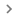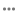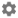# 设置 Gboard

## 下载 Gboard

• 在 Android 手机或平板电脑上安装 Gboard
• 在某些 Android 设备上，Gboard 已经是默认键盘。要确保您的设备上安装的是最新版本，请检查更新

## 设置键盘选项

1. 在 Android 手机或平板电脑上，随意打开一个可以输入文字的应用，例如 Gmail 或 Keep。
2. 点按可以输入文字的区域。
3. 点按键盘左上角的“打开功能菜单”图标4. 依次点按“更多”图标“设置”图标5. 选择要开启的设置，例如滑行输入文字更正语音输入

1. 在 Android 手机或平板电脑上，随意打开一个可以输入文字的应用，例如 Gmail 或 Keep。
2. 点按可以输入文字的区域。
3. 点按键盘左上角的“打开功能菜单”图标4. 依次点按“更多”图标“设置”图标5. 点按搜索
6. 开启显示“搜索和其他功能”按钮

1. 在 Android 手机或平板电脑上，随意打开一个可以输入文字的应用，例如 Gmail 或 Keep。
2. 点按可以输入文字的区域。
3. 点按键盘左上角的“打开功能菜单”图标4. 依次点按“更多”图标“设置”图标语言
5. 选择所需语言。
6. 选择要使用的布局。
7. 点按完成

• 阿布哈兹语
• 阿布朗语
• 亚齐语
• 阿乔利语（乌干达）
• 阿贾米语
• 阿迪格语
• 阿法尔语
• 南非语
• 阿希拉尼语
• 阿坎语
• 阿库色语
• 阿拉斯语（印度尼西亚）
• 阿尔巴尼亚语
• 阿尔巴尼亚语（托斯克方言）
• 阿勒曼尼语/瑞士德语
• 阿尔萨斯语
• 阿卢尔语
• 安汶马来语
• 阿姆哈拉语
• 阿美语（台湾）
• 阿南格语（尼日利亚）
• 阿拉伯语
• 阿拉伯语（阿尔及利亚）
• 阿拉伯语（巴林）
• 阿拉伯语（海湾）
• 阿拉伯语（利比亚）
• 阿拉伯语（摩洛哥）
• 阿拉伯语（阿曼）
• 阿拉伯语（苏丹）
• 阿拉伯语（突尼斯）
• 阿拉贡语
• 亚美尼亚语
• 亚美尼亚语（音译）
• 阿萨姆语
• 阿萨姆语（拉丁文）
• 亚述语（叙利亚）
• 亚述新亚拉姆语（伊拉克）
• 亚述新亚拉姆语（西亚）
• 阿斯图里亚斯语
• 阿瓦尔语
• 阿瓦德语
• 阿瓦德语（音译）
• 艾马拉语
• 阿塞拜疆语（阿塞拜疆）
• 阿塞拜疆语（伊朗）
• 阿塞拜疆语（俄罗斯）
• 巴哥里语
• 巴哥里语（音译）
• 巴格里语（印度）
• 巴格里语（巴基斯坦）
• 巴格里语（音译）
• 巴哈马克里奥尔语
• 巴厘语（巴厘文）
• 巴厘语（拉丁文）
• 巴尔干嘎嘎乌兹土耳其语
• 巴尔干罗姆语
• 巴尔干罗姆语（马其顿）
• 俾路支语
• 巴尔蒂语
• 班巴拉语（拉丁文）
• 班巴拉语（西非书面字母）
• 邦盖语
• 邦加马来语
• 班查语
• 班尤马语
• 巴乌雷语
• 巴萨语
• 巴什基尔语
• 巴斯克语（西班牙）
• 巴塔克曼特宁语
• 巴塔克西马隆贡语*
• 巴塔克托巴语
• 巴伐利亚语
• 白俄罗斯语
• 本奇语
• 孟加拉语（孟加拉）
• 孟加拉语（印度）
• 孟加拉语（印度、音译）
• 孟加拉语（拉丁文）
• 贝罗姆语
• 巴达维语
• 比尔语（梵文）
• 博杰普尔语（印度）
• 博杰普尔语（动态）
• 比什奴普莱利亚语
• 比什奴普莱利亚-曼尼浦尔语（音译）
• 比斯拉马语
• 博多语（孟加拉文、动态）
• 博多语（孟加拉文、音译）
• 博多语（梵文、动态）
• 博多语（梵文、音译）
• 博多语（拉丁文）
• 波斯尼亚语
• 波斯尼亚语（西里尔文）
• 布依语
• 布拉灰语（阿拉伯文）
• 布拉灰语（拉丁文）
• 布列塔尼语
• 布吉语
• 布吉语（隆塔拉文）*
• 布库苏语
• 保加利亚语
• 布卢-贝内语（喀麦隆）
• 班德利语
• 班德利语（音译）
• 缅甸语
• 布里亚特语（蒙古）
• 布里亚特语（俄罗斯）
• 喀麦隆皮钦语
• 粤语
• 佛得角克里奥尔语*
• 卡皮兹农语
• 加泰罗尼亚语
• 塞巴拉塞努福语
• 宿务语
• 中比科尔语
• 查莫罗语
• 查瓦卡诺语
• 车臣语
• 切罗基语
• 切罗基语（大小写）
• 恰蒂斯加尔语（印度）
• 恰蒂斯加尔语（动态）
• 奇加语
• 钦博拉索高地克丘亚语
• 中文（简体）
• 中文（繁体）
• 吉大港语
• 乔克托语（美国）
• 乔克维语（安哥拉）
• 乔克维语（刚果民主共和国）
• 古教会斯拉夫语
• 楚瓦什语
• 古典叙利亚语
• 科普特语*
• 康沃尔语
• 科西嘉语
• 克里语（拉丁文字）*
• 克里语（音节文字）*
• 克里米亚鞑靼语
• 克里米亚鞑靼语（拉丁文）
• 克罗地亚语
• 捷克语
• 达加拉语
• 达戈姆巴语（加纳）
• 达基尼语（印度）
• 丹语
• 当梅语
• 丹麦语
• 达尔格瓦语
• 达利语（阿富汗）
• 达萨语
• 德干语
• 迪维希语
• 敦达语
• 南扎扎其语
• 多格来语（阿拉伯文）
• 多格来语（阿拉伯文、音译）
• 多格来语（梵文）
• 多格来语（梵文、音译）
• 多格来语（拉丁文）
• Dotyali（尼泊尔）
• 东干语
• 杜里语
• 荷兰语
• 荷兰语（比利时）
• 宗卡语*
• 东法兰克语（德国）
• 东占语（拉丁文）
• 闽东语
• 东奥罗莫语（埃塞俄比亚）
• 东达芒语（尼泊尔）
• 埃比拉语
• 埃菲克语
• 艾米利亚语
• 英语（澳大利亚）
• 英语（加拿大）
• 英语（英国）
• 英语（印度）
• 英语（肯尼亚）
• 英语（尼日利亚）
• 英语（菲律宾）
• 英语（南非）
• 英语（美国）
• 厄尔兹亚语
• 世界语
• 爱沙尼亚语
• 埃维语*
• 埃翁多语
• 埃斯特雷马杜拉语
• 芳语
• 芳蒂语
• 法罗语
• 斐济印地语（梵文）
• 斐济印地语（拉丁文）
• 斐济语
• 菲律宾语
• 芬兰语
• 丰族语
• 法语
• 法语（非洲）
• 法语（比利时）
• 法语（加拿大）
• 法语（瑞士）
• 弗里西语
• 弗留利语
• 富拉语（阿德拉姆文）
• 富拉语（拉丁文）
• 富拉尼语（阿德拉姆文）*
• 富拉语（拉丁文）*
• 嘎嘎乌兹语
• 加利西亚语（西班牙）
• 嘉华语（印度）
• 嘉华语（动态）
• 加罗语（孟加拉语）
• 加罗语（拉丁文）
• 迦约语（印度尼西亚）
• 吉兹语
• 格鲁吉亚语（格鲁吉亚文）
• 格鲁吉亚语（教士体）
• 德语
• 德语（奥地利）
• 德语（比利时）
• 德语（卢森堡）
• 德语（瑞士）
• 加纳皮钦语
• 盖格阿尔巴尼亚语
• 吉拉克语
• 吉尔伯特语
• 吉利亚马语（肯尼亚）
• 哥达瓦里语（印度）
• 戈戈语（坦桑尼亚）
• 冈德语（梵文）
• 冈德语（泰卢固文）
• 哥伦打洛语（印度尼西亚）
• 希腊语
• 希腊语（塞浦路斯）
• 希腊语（本都）
• 希腊语（音译）
• 格陵兰语
• 瓜德罗普克里奥尔语
• 瓜拉尼语
• 圭亚那克里奥尔语
• 几内亚比绍克里奥尔语
• 古吉拉特语
• 古吉拉特语（拉丁文）
• 古吉拉特语（音译）
• 古贾尔语（印度）
• 古贾尔语（巴基斯坦）
• 古拉尼语
• 古西语（肯尼亚）
• 圭亚那克里奥尔语
• 豪萨语（坦桑尼亚）
• 海地克里奥尔语
• 哈卡钦语
• 客家语
• 亥比语（梵文）
• 亥比语（奥里亚文）
• 哈尼语
• 哈劳提语
• 哈里亚纳语（印度）
• 哈里亚纳语（动态）
• 豪萨语
• 豪萨语（加纳）
• 夏威夷语
• 夏威夷皮钦语
• 哈乌语
• 哈亚语（坦桑尼亚）
• 希伯来语
• 赫赫语（坦桑尼亚）
• 希利盖农语
• 山地马里语
• 印地语
• 印地语（拉丁文）
• 印地语（音译）
• 希里摩图语
• 苗语
• 苗语（中国）
• 苗语（中国）
• 匈牙利语
• 洪斯吕克语
• 伊班语
• 伊比比奥语
• 冰岛语
• 伊博语
• 伊洛果语
• 印度尼西亚语
• 印度科希斯坦语
• 英古什语
• 国际语
• 国际音标*
• 因纽特语（拉丁文字）*
• 因纽特语（音节文字）*
• 爱尔兰语
• 南恩德贝莱语
• 伊索科语
• 意大利语
• 意大利语（瑞士）
• Itawit 语
• 勉语
• 牙买加语
• 占碑马来语
• 日语
• 爪哇语
• 爪哇语（爪哇字母）
• 景颇语
• 迪尤拉语*
• 基切语
• 卡巴尔达语
• 卡达山-杜顺语（马来西亚）
• 格陵兰语
• 卡拉姆科希斯坦语
• 卡尔梅克语
• 卡姆巴语
• 坎巴塔语（吉兹语）
• 坎巴塔语（拉丁文）
• 坎那达式英语
• 康格里语
• Kankanaey 语
• 卡纳达语
• 卡纳达语（拉丁文）
• 卡纳达语（音译）
• 卡瑙杰语
• 卡努里语
• 邦板牙语
• 卡拉恰伊语
• 卡拉卡尔帕克语（西里尔文）
• 卡拉卡尔帕克语
• 基那来阿语（菲律宾）
• 卡累利阿语
• 卡罗语
• 克什米尔语（阿拉伯文）
• 克什米尔语（阿拉伯文、音译）
• 克什米尔语（梵文）
• 克什米尔语（梵文、音译）
• 克什米尔语（拉丁文）
• 卡舒比语（波兰）
• 哈萨克语
• 吉打马来语
• 凯克其语
• 吉兰丹-北大年马来语（阿拉伯文）
• 卡西语
• 高棉语（柬埔寨）*
• 霍拉桑突厥语
• 霍拉桑突厥语（阿拉伯文）
• 科瓦语
• 吉库尤语
• 金邦杜语（安哥拉）
• 卢旺达语
• 基普西吉语
• 北扎扎其语（土耳其）
• 吉土巴语
• 廓克博若克语（孟加拉文）
• 廓克博若克语（拉丁文）
• 科隆语（德国）
• 科梅灵语
• 科米语
• 科米语（俄罗斯）
• 科米-齐良语
• 刚果语
• 贡根语
• 贡根语（梵文）
• 贡根语（卡纳达语）
• 贡根语（拉丁文）
• 贡根语（音译）
• 康孔巴语
• 孔索语
• 韩语
• 科尔库语（印度）
• 科罗纳达尔-布拉安语
• 克里奥尔语
• 库伊语
• 库矛语
• 库矛语（印度）
• 库梅克语
• 库尔德语
• 库尔德语（伊朗）
• 库尔德语（伊拉克，拉丁文）
• 库鲁克语
• 库台马来语
• 喀奇语（印度）
• 库瓦亚马语
• 吉尔吉斯语
• 拉迪诺语
• 拉迪诺语（以色列）
• 拉克语
• 拉基语
• 拉科塔语
• 拉玛语
• 兰巴迪语（梵文）
• 兰巴迪语（卡纳达语）
• 兰巴迪语（泰卢固文）
• 楠榜语（印度尼西亚）
• 兰戈语
• 老挝语*
• 拉特加莱语
• 拉丁语
• 拉脱维亚语
• 勒多-凯利语
• 伦杜语
• 雷布查语*
• 列兹金语（阿塞拜疆）
• 列兹金语（俄罗斯）
• 利古里亚语
• 林布语
• 林布语*
• 林堡语
• 林格拉语
• 立陶宛语
• 利沃尼亚语
• 李维卡累利阿语
• 逻辑语
• 伦巴第语
• 低地德语
• 下索布语
• 卢巴-加丹加语
• 卢干达语
• 卢格巴拉语
• 卢奥语
• 鲁沙伊语
• 卢森堡语
• 玛安延语（印度尼西亚）
• 马塞族语
• 马耶马耶语
• 马其顿语
• 马都拉语
• 摩揭陀语
• 摩揭陀语（印度）
• 马京达瑙语
• 迈蒂利语
• 迈蒂利语（拉丁文）
• 迈蒂利语（音译）
• 望加锡马来语
• 望加锡语（印度尼西亚）
• 马孔德语
• 马尔加什语
• 马来语（文莱）
• 马来语（中部）
• 马来语（马来西亚）
• 马来语（阿拉伯文）
• 马来语（阿拉伯文）（文莱）
• 马来语（拉丁文）（新加坡）
• 马拉雅拉姆语（印度）
• 马拉雅拉姆语（拉丁文）
• 马拉雅拉姆语（音译）
• 马耳他语
• 马尔维语
• 马马萨语
• 万鸦老马来语（印度尼西亚）
• 曼迪安语
• 曼迪阿里语
• 曼丁哥族语
• 芒加莱语
• 马来西亚式英语
• 曼尼普尔语（孟加拉文）
• 曼尼普尔语（孟加拉文、音译）
• 曼尼普尔语（拉丁文）
• 曼尼普尔语（梅泰文）
• 曼尼普尔语（梅泰文、音译）
• 马恩岛语
• 毛利语
• 马拉瑙语
• 马拉地语（印度）
• 马拉地语（拉丁文）
• 马拉地语（音译）
• 马尔瓦里语（印度）
• 马尔瓦里语（巴基斯坦）
• 马萨巴语
• 马赞德兰语
• 草原马里语
• Mehri 语
• 马兰诺语
• 门德语
• 梅鲁语（肯尼亚）
• 马尔瓦里语（印度）
• 梅瓦里语
• 闽南语
• 米南佳保语
• 明格列尔语
• Moba 语
• 莫克沙语
• 蒙古语
• 蒙贡多语
• 黑山语（西里尔文）
• 黑山语（拉丁文）
• 莫西语
• 穆纳语
• 蒙当语*
• 蒙达里语（孟加拉）
• 蒙达里语（拉丁文）
• 蒙达里语（奥里亚文）
• 芒语（越南）
• 穆西语（印度尼西亚）
• 纳瓦特尔语
• 楠德语
• 南迪语
• 瑙鲁语*
• 纳瓦霍语
• 恩道语
• 恩敦加语
• 那不勒斯语
• 尼泊尔语（梵文、动态）
• 尼泊尔语（拉丁文）
• 尼泊尔语（尼泊尔）
• 尼泊尔语（音译）
• 尼瓦尔语（动态）
• 恩加朱语（印度尼西亚）
• 格巴亚恩巴卡语
• 尼亚斯语
• 尼日利亚皮钦语
• 尼马地语
• 纽埃语
• 恩科勒语（乌干达）
• 特尔纳特马来语（印度尼西亚）
• 北贝齐米萨拉卡-马拉加斯语
• 北欣德科语
• 北卢尔语
• 闽北语
• 北恩德贝莱语
• 北萨米语
• 北索托语
• 挪威语
• 挪威语（波克默尔文）
• 恩查姆语
• 努佩语
• 尼亚库萨语
• 尼扬韦齐语（坦桑尼亚）
• 尼扬贾语
• 尼亚图鲁语（坦桑尼亚）
• 尼奥罗语
• 奥克西当语
• 奥里亚语
• 奥里亚语（拉丁文）
• 奥里亚语（音译）
• 奥罗莫语
• 欧塞奇语*
• 奥塞梯语
• 巴奥克伦语*
• 帕哈里-波特瓦里语
• 帕莫纳语
• 邦阿西楠语
• 帕皮阿门托语
• 巴布亚马来语
• 帕尔卡里科里语
• 普什图语
• 牙买加土语
• 波斯语（阿富汗）
• 波斯语（伊朗）
• 普法尔茨德语
• 庇卡底语
• 皮埃蒙特语
• 平原马拉加斯语
• 波科特语
• 波兰语
• 葡萄牙语
• 葡萄牙语（非洲）
• 葡萄牙语（安哥拉）
• 葡萄牙语（巴西）
• 葡萄牙语（澳门）
• 葡萄牙语（葡萄牙）
• 旁遮普语（阿拉伯文）
• 旁遮普语（阿拉伯文、音译）
• 旁遮普语（果鲁穆奇文）
• 旁遮普语（果鲁穆奇文、音译）
• 旁遮普语（拉丁文）
• 卡什加语
• 克丘亚语
• 克丘亚语（阿亚库乔）
• 克丘亚语（库斯科）
• 朗布尔语
• 朗布尔语（梵文）
• 留尼汪克里奥尔语
• 林科纳达比考尔语
• 罗马尼亚语
• 罗马尼亚语（摩尔多瓦）
• 罗曼什语
• 隆迪语
• 俄语
• 俄语（白俄罗斯）
• 俄语（吉尔吉斯斯坦）
• 卢森尼亚语
• 沙巴马来语
• 萨德里语
• 圣卢西亚克里奥尔法语
• 萨卡拉瓦-马拉加斯语
• 萨摩亚语
• 萨莫吉提亚语
• 桑戈语
• 梵语
• 梵语（拉丁文）
• 梵语（音译）
• 桑塔利语（孟加拉文）
• 桑塔利语（梵语）
• 桑塔利语（拉丁文）
• 桑塔利语（欧甘文）
• 桑塔利语（欧甘文、音译）
• 西莱基语（乌尔都语/阿拉伯语）
• 撒丁尼亚语
• 萨萨克语（印度尼西亚）
• 萨沙里语（意大利）
• 苏格兰语
• 苏格兰盖尔语
• 塞巴古拉格语
• 塞尔维亚语
• 塞尔维亚语（黑山，西里尔文）
• 塞尔维亚语（塞尔维亚，西里尔文）
• 塞尔维亚语（拉丁文）
• 塞舌尔克里奥尔法语
• 塞索托语
• 塞索托语（莱索托）
• 斯高克伦语
• 沙卡瓦蒂语
• 辛那语（巴基斯坦）
• 修纳语
• 西伯利亚鞑靼语
• 西西里语
• 西里西亚语
• 西马隆贡语
• 信德语（阿拉伯文）
• 信德语（阿拉伯文、音译）
• 信德语（梵文、动态）
• 信德语（梵文、音译）
• 信德语（拉丁文）
• 僧伽罗语
• 斯洛伐克语
• 斯洛文尼亚语
• 索加语
• 索马里语
• Songe 语（刚果民主共和国）
• 南玻利维亚克丘亚语*
• 南欣德科语
• 南库尔德语
• 南卢尔语
• 西班牙语（阿根廷）
• 西班牙语（拉丁美洲）
• 西班牙语（墨西哥）
• 西班牙语（西班牙）
• 西班牙语（美国）
• 苏里南语*
• 苏库马语
• 巽他语
• 巽他语（阿拉伯文）
• 巽他语（拉丁文）
• Supyire 语
• 素拉杰普尔语
• 斯瓦希里语
• 斯瓦特语
• 斯威士语（南非）
• 瑞典语
• 瑞典语（芬兰）
• 塞海蒂语
• 塞海蒂语（塞洛提纳格瑞文）
• 塞海蒂语（拉丁文）
• 塔巴萨兰语（俄罗斯）
• 塔加路语
• 塔希提语
• 黑傣语（拉丁文）
• 黑傣语（越南傣文）*
• 傣仂语（西双版纳新傣文）
• 塔吉克语
• 塔里什语（阿塞拜疆）
• 塔里什语（伊朗）
• 塔里什语（俄罗斯）
• 塔马齐格特语（提非纳文）*
• 塔马齐格特语*
• 泰米尔语（印度）
• 泰米尔语（印度、音译）
• 泰米尔语（拉丁文）
• 泰米尔语（新加坡）
• 泰米尔语（斯里兰卡）
• 鞑靼语
• 塔特语
• 陶苏格语
• 岱语
• 泰卢固语（印度）
• 泰卢固语（拉丁文）
• 泰卢固语（音译）
• 提姆语
• 特索语
• 德顿语
• 泰语
• 藏语*
• 蒂格尼亚语
• 提夫语
• 巴布亚皮钦语
• 托克劳语
• 汤加语
• 土柔语（乌干达）
• 托拉查语
• 托尔瓦里语
• 特立尼达克里奥尔英语
• 文达语
• 宗加语
• 茨瓦纳语
• 图鲁语（音译）
• 图鲁语（印度）
• 图尔卡纳语（肯尼亚）
• 土耳其语
• 土耳其语（塞浦路斯）
• 土库曼语
• 图瓦语
• 乌德穆尔特语
• 乌克兰语
• 姆班杜语（安哥拉）
• 上撒克逊语（德国）
• 上索布语
• 乌尔都语（印度）
• 乌尔都语（拉丁文）
• 乌尔都语（巴基斯坦）
• 乌尔都语（音译）
• 乌鲁姆语
• 维吾尔语
• 乌兹别克语（西里尔文字）
• 乌兹别克语（拉丁文字）
• 瓦伊语*
• 瓦萨维语
• 瓦萨维语（古吉拉特文）
• 威尼斯语
• 外普西亚语（外坡思语）
• 越南语
• 夫拉克斯罗姆语
• 夫拉克斯罗姆语（罗马尼亚）
• 佛罗语
• 瓦格迪语
• 瓦隆语
• 瓦济里语
• 瓦瑞语
• 瓦尤语
• 威尔士语
• 西佛兰德语
• 西弗里西亚语
• 西克耶语（克耶黎文）
• 沃洛夫语
• 科萨语
• 湘西苗语
• 雅库特语
• 瑶语
• 意第绪语
• 意第绪语（以色列）
• 邕北壮语（中国）
• 约鲁巴语
• 右江壮语
• 尤卡坦玛雅语（墨西哥）
• 扎扎语
• 扎扎其语
• 西兰语
• 壮语
• 祖鲁语